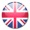JGMsmart.TC - Type & Calculate - is a simple calculator wich does not require the complex surface of a conventional calculator. Die zu berechnende Formel wird einfach eingegeben und mit einem Tastendruck berechnet. The formula to be calculated is simply entered and calculated with a keystroke.

This manual describes version 1.1.0 of JGMsmart.TC. Earlier version may have less features.

JGMsmart.TC can be accessed at any tima via an icon in the taskbar that is always just a click away.

JGMsmart.TC supports the following functions:

Operators: + - * / ^ ( ) %

Functions: abs, sqrt, cbrt, sin, cos, tan, sinh, cosh, tanh, ln, log2, log10, deg, rad, n!, floor, ceil, round

With these functions you can create complex formulas that can be stored for later use. So you can create your own formula collection.

You can use constants in the calculations that can be added to the formula by a click.

You can use constants in the calculations that can be added to the formula by a click.

Predefined are following constants:

Pi - Archimedes constant

e - Eulers number

sqrt2 - Pythagoras constant, square root of 2

g - Acceleration due to gravity

G - Newtonian constant of gravitation

c - Speed of light

kB - Boltzmann constant

Other constants can easily be added.

The calculation result can be manually or automatically copied to the pasteboard and is thus available in other applications.

Information